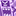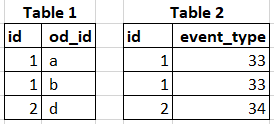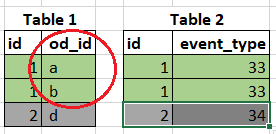# App Development

Announcements
Join “Do More With Qlik” with Mike Tarallo to get a first-hand look at the New Authoring Experience, June 29th: REGISTER HERE
cancel
Showing results for
Did you mean:Contributor II

## COUNT(IF()) vs COUNT({<>})

Hello Community,

I have a big question since it is drawling some big discrepancies in my thought process.

I am trying to count with a condition...

I am under the impression I should be able to do this with either of the two lines of code listed below; however, I get two different results. So my question is how do the two lines of code act differently? Or is there something being done wrong on the back end that would have caused this.

Count({<event_type={33,34}>}od_id)

vs

Count(if(event_type=33, od_id) or if(event_type=34, od_id) )

Note I have tried different variations of these.

I have also tried to diagnose this with only one event_type and still get two different results.

Count({<event_type={33}>}od_id)      //will produce 102.1K

vs

Count(if(event_type=33, od_id) )        // will produce 173.6K

Clarification on how these two formulas are working differently would be greatly appreciated.

Labels (4)

• ### functionCreator III

Hi,

Count({SetAnalysis}) = Override the current selection before evaluating the Count expression. This filter action is applied to the whole data model, not row-by-row

Count(IF()) = Evaluate the IF condition in each row, then apply the Count() function on top of the resulting dataset.

For example, let's say you have the following data model with 2 tables. Note that in Table 2 value (1,33) is duplicated.1. Count({<event_type={33}>}od_id) = 2

• First Qlik Sense makes a selection on event_type=33 (on top of current selection if any)
• Then it performs a count on possible values of od_id, which returns 22. Count(if(event_type=33, od_id) )  = 4

• First Qlik Sense performs a natural join on tables containing event_type and od_id. Because (1,33) is duplicated in Table 2, it is joined twice.
• In this joined table, condition if(event_type=33, od_id) is evaluated for each row.
• Finally, Count is evaluated on results of the if condition (including the null values) , which returns 4As a thumb rule, if condition should be used when evaluation needs to be done in a row-by-row basis. Otherwise it's better with Set Analysis.

Hope this helps!

BR,

Vu Nguyen